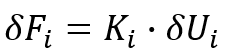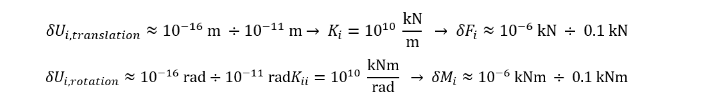# Convergence criterias

Convergence criterias are limit relations prescribed for some error values, adjustable on the ‘Nonlinear static analysis’ dialogue.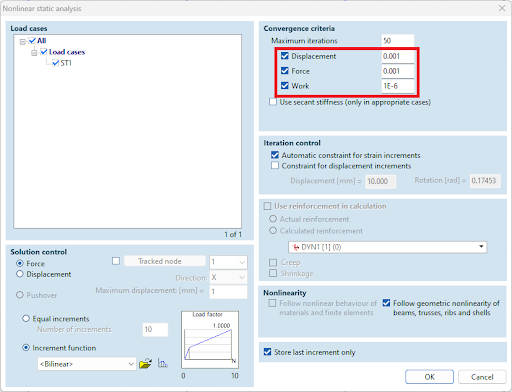# Types of convergence criteria#

## Convergence in displacement#

For example, let’s look at the last iteration in the second load increment, between the light blue and the orange circles. Let the displacement in the last iteration be denoted by delta U. Moreover, let the total displacement measured from the unloaded case denoted by UIn MDOF case, these vectors have a scalar component for each degree of freedom. The relative error in displacement is defined as the quotient of the norms of these vectors.

In other words, this error value describes how much the whole structure moved in the last iteration compared to the total displacement measured from the unloaded state.

Definition of the relative error in displacement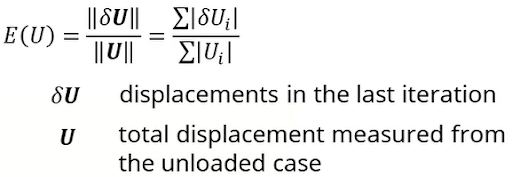This type of convergence error can also be visualized both in the state space and on the deformed shape of the model. AxisVM uses the so-called “taxicab” norm, which is the sum of the absolute value of the vector components instead of the length of the vector.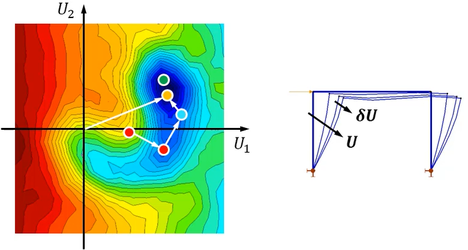## Convergence in force#

Let delta F denote the difference between the applied load and the force response of the structure at the end of the last iteration. It is the unequilibrated or residual force.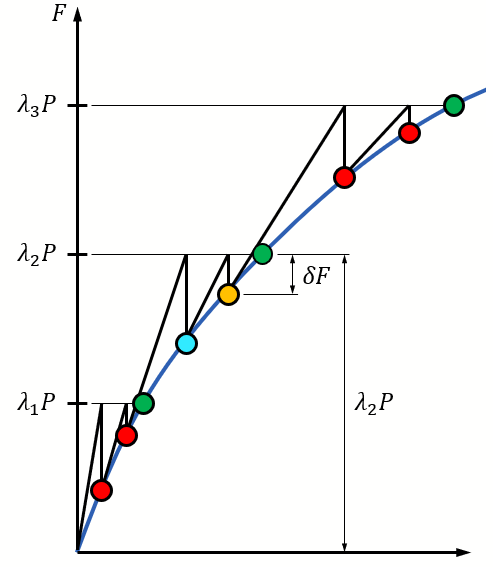It is the force with which the equilibrium is violated. Moreover, let the applied load at the current load level be denoted by lambda times P. In MDOF case, these vectors also contain a scalar component for each degree of freedom. The relative error in force is defined as the quotient of the norms of these vectors.

In other words, this error value describes how much the force equilibrium is violated for the whole structure.

Definition of the relative error in force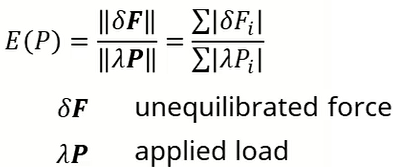## Convergence in work#

The nominator of the formula is the work done by the unequilibrated forces on the displacements in the last iteration. Its denominator is the work done by the applied forces on the total displacement measured from the unloaded state. It is a mixed quantity of forces and displacements.

Definition of the relative error in work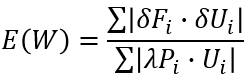## Fulfilment of convergence criteria#

When a convergence criterion is fulfilled, it means that the error summarized for the entire model is small enough.

Displacement convergence means the whole structure moved small enough in the last iteration.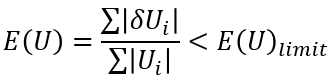Force convergence means that the sum of the unequilibrated forces for the whole structure is small enough.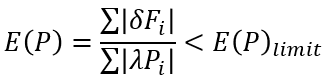Work convergence means that the work done by the unequilibrated forces on the iterational displacements for the whole structure is small enough.

Which one is recommended to turn on, and in which cases?

• Displacement criterion is always suggested to be turned on, as the displacements are the primary variables. Its default value is 0.001, which is in most cases, tight enough for accurate results. If the convergence is almost but not achieved, it is suggested to loosen the limit to 0.01. It eventuates inaccurate displacements, but we can see an approximate deformed shape that can help to find the reason of the weak convergence. This trick can often be applied to models with RC.
• Force criterion is also recommended to turn on with a default value of 0.001, especially if accurate internal forces or support reactions are necessary. This is the case if member design based on internal forces will be performed. If the nodal force equilibrium is violated, tightening the limit to 0.0001 (1e-4) or 0.00001 (1e-5) is recommended.

Limitation of accuracy in displacement and in force

What does the fulfilment of convergence criteria means precisely?

Their statements apply to the whole structure, but their fulfilment says nothing about the distribution and especially about the maximum of the error portions belonging to the individual DOFs.

The force criterion means that although the convergence criterion is fulfilled, the nodal force equilibrium may be highly violated at some individual DOFs. Nodes with stiff supports are the most concerned.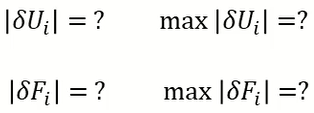The reason for this is as follows. The primary variables are the displacement components. These are calculated directly from the system of equilibrium equations. There is a numerical limit to the accuracy of them. This is small enough to be negligible in the displacement results, but the high stiffness of rigid support can magnify this small inaccuracy, which can lead to the violation of force equilibrium. Therefore, for nonlinear analysis, the stiffness value of supports considered rigid would be necessary to decrease from its default value.